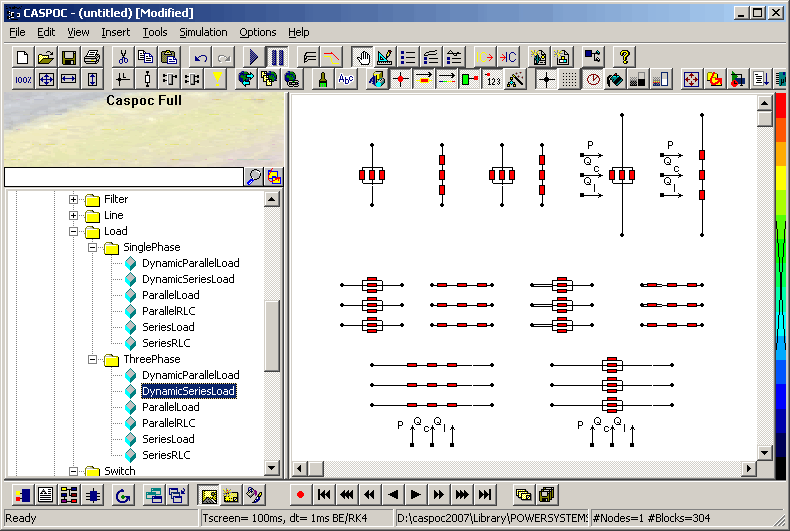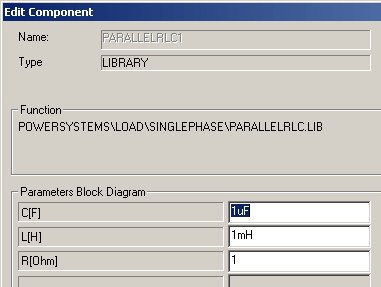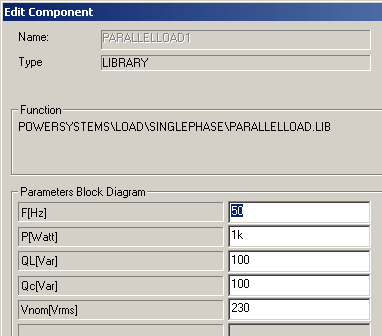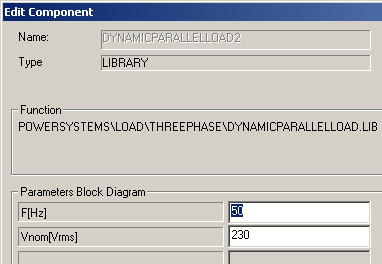• Introduction
• What is in this manual
• What is Caspoc
• User interface
• Introduction
• Starting
• Simulation
• Editing
• Viewing and printing
• Getting Started
• Basic editing
• Simulation in the time domain
• Basic User Interface Topics
• Editing
• Simulation
• Viewing
• Library
• Reports
• Project management
• Circuit and Block Diagram Components
• Introduction
• Cscript and user defined functions
• Component parameters
• Modeling Topics
• Introduction
• Power Electronics
• Semiconductors
• Electrical Machines
• Electrical drives
• Power Systems
• Mechanical Systems
• Thermal Systems
• Magnetic Circuits
• Green Energy
• Coupling to FEM
• Experimenter
• Analog hardware description language
• Embedded C code Export
• Coupling to Spice
• Small Signal Analysis
• Matlab coupling
• Tips and tricks
• Appendices

## Parallel and Series Load and RLC Branches.

There are several series and parallel combinations of RLC components to create electrical loads, or to be used as series and/or parallel branches. The dynamic models are convenient when you want to specify the active and reactive power of the load, without specifying the RLC parameters yourself.All blocks are supplied as single and three phase models.

### Parallel and Series Branch

Parallel branch The Parallel RLC branch block implements a linear load as a parallel combination of RLC elements. The values of R, C and L have to be specified.

Series branch The Series RLC Branch block implements a linear branch as a series combination of R L C elements. The values of R, C and L have to be specified.

### Branch parametersBranch Parameters
 Parameter Default Function R 1[Ohm] Branch resistance. L 1m[Henry] Branch inductance. C 1u[Farad] Banch capacitance.

Parallel load The Parallel RLC Load block implements a linear load as a parallel combination of RLC elements. At the specified frequency, the load will exhibit a constant impedance and its power will be proportional to the square of the applied voltage.

Series load The Series RLC Load block implements a linear load as a series combination of R L C elements. At the specified frequency, the load will exhibit constant impedance and its power will be proportional to the square of the applied voltage.Parameter Default Function F 50[Hz] The nominal frequency, in Hertz (Hz). P 1000[Watt] The active power of the load, in watts. QL 100[Var] The inductive reactive power QL, in vars. Specify a positive value, or zero. QC 100[Var] The capacitove reactive power QC, in vars. Specify a positive value, or zero. Vn 230[Vrms] The nominal voltage of the load, in volts rms (Vrms).

### Dynamic Parallel and Series Load

The difference with the previous parallel and series load is that the active and reactive power can be controlled. The values for the active and reactive power have to be connected to the appropriate inputs of the blocks.

Parallel load The Parallel RLC Load block implements a linear load as a parallel combination of RLC elements. At the specified frequency, the load will exhibit a constant impedance and its power will be proportional to the square of the applied voltage.

Series load The Series RLC Load block implements a linear load as a series combination of R L C elements. At the specified frequency, the load will exhibit constant impedance and its power will be proportional to the square of the applied voltage.